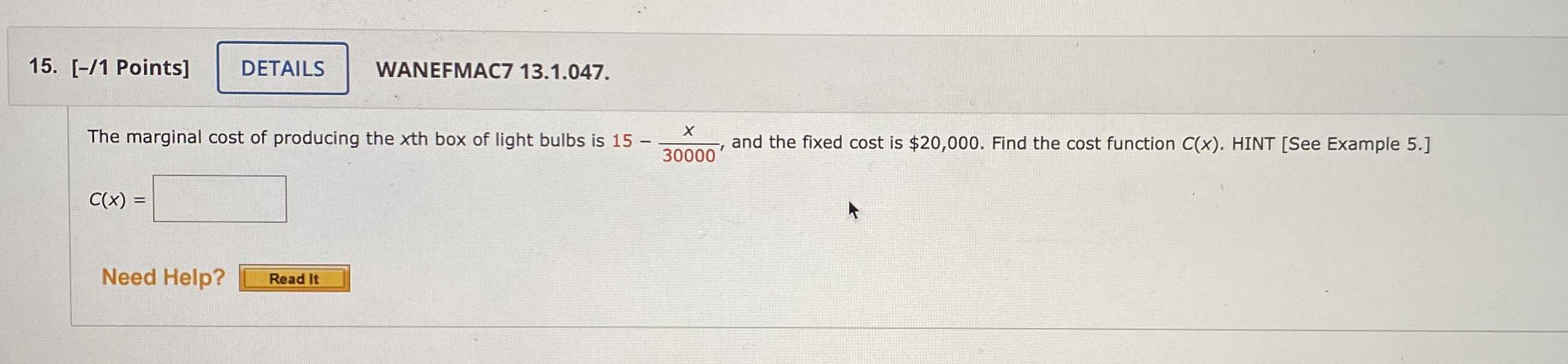### ¿Todavía tienes preguntas de matemáticas?

Pregunte a nuestros tutores expertos
Algebra
Pregunta15.The marginal cost of producing the xth box of light bulbs is $$15 - \frac { x } { 30000 }$$ , and the fixed cost is $$\ 20,000$$ . Find the cost function $$C ( x )$$ .

$$C ( x ) =$$

$$- \frac{x^2}{60000}+ 15x+ 20000$$